Document Type : Original Research Article

Author

Department of Chemical Engineering, Mahshahr Branch, Islamic Azad University, Mahshahr, Iran

Abstract

Almost all recent activities on the prediction of hydrate crystal formation conditions are based on the science of thermodynamics. In the study of equilibrium in the formation of hydrates, we are faced with different phases, and therefore the best way is to use the specific model of that phase for each phase so that we can calculate the fugacity’s more accurately. The most common thermodynamic model used to predict the properties of a gas phase is the "state equation". In the hydrate system, two types of additives are used. When the hydrate phenomenon is known to be harmful, inhibitors are used to prevent the formation of hydrates. In addition, due to the unique properties of hydrates, improvers are used to improve the conditions for hydrate formation. Thermodynamic models in the presence of inhibitors are modified by calculating the coefficient of activity and solubility of the components. Because in the presence of additives, the activity coefficient will no longer be one and on the other hand in the presence of inhibitors the solubility of gaseous components in the aqueous phase changes. The paper examines the model and its results in the presence of inhibitors. The inhibitors considered in this work are ethylene glycol and methanol and the prediction of hydrate formation in the presence of this type of inhibitor with state equations such as SRK and PR has been investigated.

Graphical Abstract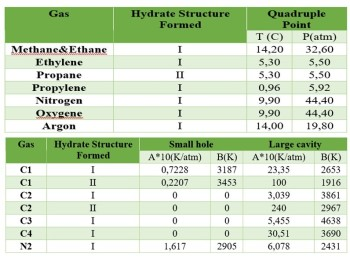Keywords

Main Subjects

###### ##### Full Text

Introduction

ne of the models that can be used for this phase due to the low solubility of hydrocarbons in water is the law of art [1-5]. According to this law, the artistic constant of component i in solvent 1 (here water) H i, 1 is independent of the composition of the mixture and is merely a function of temperature and a weak function of pressure. Artistic constant thermodynamic definition is: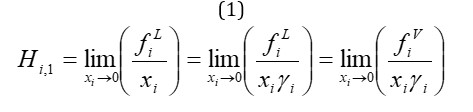Where fiL fugacity is in the liquid phase, xi is the molar fraction and γi are the activity coefficient of component i in the liquid phase, and fiV fugacity is component i in the vapor phase [6-9]. If the solubility is very small (xi << 1) we can write with a good approximation: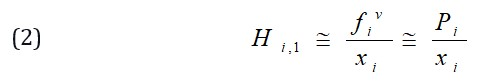Pi is the partial pressure of component i in the gas phase. If the partial pressure of component i is equal to one atmosphere, the dissolution rate of component i is obtained from the following relation: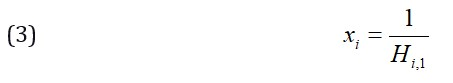The effect of pressure on an artistic constant is usually negligible, but the effect of pressure can be obtained by the following relation called Kasarnovsky - Krichevsky: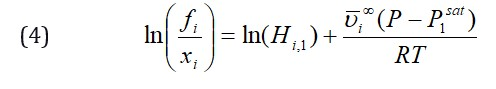vi is the molar fractional volume of component i at infinite dilution and P1sat is the saturated vapor pressure of the solvent (water) [10-15]. Because it is difficult to pinpoint the value of vi for different working gases, the value of vi = 35 cc / mol is usually used for light hydrocarbons [16-19].

Hydrate phase

All available models use the modified Vandwalls-Plateau model "VDW-P" to predict the hydrate phase properties.

The assumptions of the VDW-P model are summarized as follows:

1- Each hole in the crystal lattice can accept a molecule as a guest.

2- The ideal gas partition function can be used for guest molecules.

3- The intermolecular forces of the guest and the water molecules are expressed by the potential function and each spherical hole is considered [20-25].

4- The forces between the guest gas molecules in the network are ignored.

5. There is a force only between the gas molecules in the cavity and the water molecules that surround it.

6. The free energy distribution of water molecules is independent of how the molecules occupy the crystal lattice [26-29].

Physical model presented by Parrish and Prausnitz

In this model, only London forces are used to describe the gas-water interaction. The difference between the chemical potential of water in the empty hydrate network (µwβ) and the hydrate phase (µwΗ) is calculated from the following equation :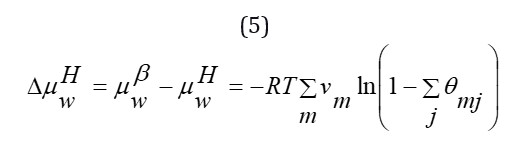vm is the number of m-type cavities per water molecule in the hydrate crystal lattice. The fraction of m-type cavities occupied by the gas compound l is defined as follows: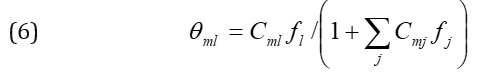Which is the Langmuir constant Cml and the fugacity of the gaseous component, which is defined as follows: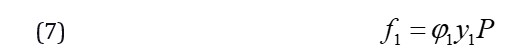But for parameter C, the following equations are given by Parish and Praznits:

(8)                            Cmj=(Amj/T)*(exp(Bmj/T))

To predict the hydrate formation conditions, the value (µwβ - µwH) must be calculated from the above equations. In equilibrium, the chemical potential of water in the hydrate phase is equal to any of the other co-living phases [30-35].

Depending on whether water or ice is available, the potential difference is defined as follows: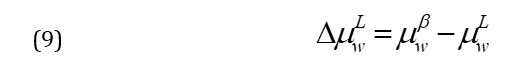The following relation is presented for the potential difference in the aqueous phase: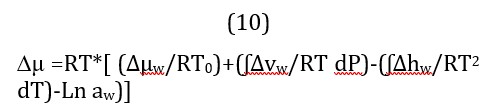μ∆ =RT*[ (∆μw/RT0)+(ʃ∆vw/RT dP)-(ʃ∆hw/RT2 dT)-Ln aw)]

In the above relation, the parameters are obtained experimentally, which is available in the references and is given in Table 1 below

Table 1. Hydrate structure and required constants 

 Structure II Structure I Property 883 1264 ∆ µw(J/mol) -5201 -4858 ∆ hw(J/mol) 5.0 4.6 ∆ vw(cm3/mol) 38.120-.0141(T-273.15) ∆ CpL(J/mol.K)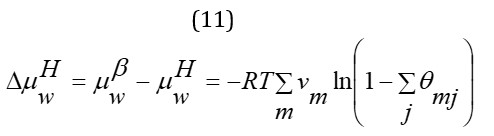Using Equation (4-11), the conditions for hydrate formation are expressed as follows:

(12)                                             ∆μ_w^L=∆μ_w^H

By solving the above relation by conjecture and error, the equilibrium pressure of hydrate formation at the desired temperature is calculated. Inhibitors such as methanol, ethanol, ethylene glycol, etc. are used in oil and gas lines to prevent the formation of hydrates . These materials alter the water activity (used above using the NRTL model). This change affects the equilibrium potential and increases the equilibrium pressure of hydrate formation. Therefore, at a certain temperature, by inserting an inhibitor in the pipeline, the pressure of hydrate formation increases and this causes the hydrate not to form at the injection pressure or gas or oil transfer. It should be noted that in this work, the activity Water in the above formula (aw) is obtained using the NRTL activity coefficient model: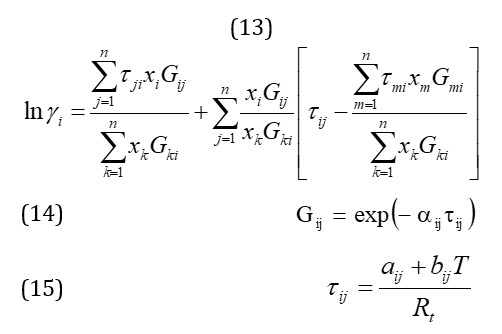The values αij, aij and bij are model parameters .

Modeling steps based on Parrish and Prausnitz algorithm

1-At the beginning of modeling, we have assumed that by having information such as temperature and type of inhibitor and its weight percentage. We intended to predict the equilibrium conditions of hydrate formation. Therefore, in the first step, according to Table (2), if the temperature is higher than the quadruple temperature, we will not have hydrate formation at all. Otherwise, we will go to the next step .

Table 2. Quadruple point temperature and pressure for pure gaseous hydrates

 Gas Hydrate Structure Formed Quadruple Point T (C) P(atm) Methane&Ethane I 14,20 32,60 Ethylene I 5,30 5,50 Propane II 5,30 5,50 Propylene I 0,96 5,92 Nitrogen I 9,90 44,40 Oxygene I 9,90 44,40 Argon I 14,00 19,80

2- In this step, we calculated the Langmuir Cm coefficient. Aij and Bij are fitted constants from laboratory data whose values are given in Table (3).

Table 3. Experimental coefficients for calculating Langmuir coefficients

 Gas Hydrate Structure Formed Small hole Large cavity A*10(K/atm) B(K) A*10(K/atm) B(K) C1 I 0,7228 3187 23,35 2653 C1 II 0,2207 3453 100 1916 C2 I 0 0 3,039 3861 C2 II 0 0 240 2967 C3 I 0 0 5,455 4638 C4 I 0 0 30,51 3690 N2 I 1,617 2905 6,078 2431

3- In the first step, as a preliminary guess, we considered the fugacity coefficient and the activity coefficient equal to one.

4- We considered the pressure according to the proposed Parish and Praznitz equation or the same equation (16) as the initial conjecture .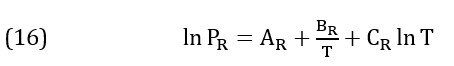The coefficients of this equation are given in Table (4).

Table 4. Determination of PR pressure prediction in the temperature range of 273 to 300 degrees Kelvin

 CR BR AR 187.719 44344.0 -1212.2 Methane

We did the calculation according to equations (5) and (10)   and  its difference E0.

Obviously, we will reach the equilibrium condition of hydrate formation when the final E equals zero .

5- In this step, to correct the coefficients of fugacity and activity with the pressure estimated in step 5 and the weight percentage of the components mentioned in step one, and also to calculate the PS from the Antoine equation mentioned in equation (17), We calculated Equation (18).

(17)                                          Log P =A-[B/(T+C)]

(18)                                             yi=( xi*ɣi*PS)/ɸi*P

7- If E1 is zero, the considered pressure will be the equilibrium pressure of hydrate formation. Otherwise, using Newton-Raphson equation, we guess a new pressure and return to step 6 and calculate a new E. These steps are repeated until the value of E or the difference of chemical potentials is equal to zero.

At low pressures, the algorithm responds quickly. Even with poor initial guesses for P, we get results with the first few iterations. At pressures above about 300 atmospheres we will respond more slowly and even need 20 to 30 repetitions .

Equations of state

In this design, third degree equations of PR and SRK are used and finally a comparison between their results is presented. These state equations are one of the most widely used equations in modeling calculations. The following section is a reference to these equations.

SRK mode equation

This equation is actually a modified form of the RK state equation proposed by Soave (1972). The term a is modified to calculate the vapor pressure of hydrocarbons using a decentralized coefficient as a correction parameter. The general form of the SRK state equation is based on the definition equation May be.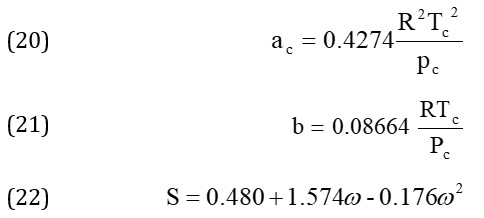Also, the compressibility coefficient is obtained by calculating the roots of the following equation.

(23)                                  Z3-Z2-Z (A-B-B2)-AB=0

The above equation has three roots, the least of which are related to the liquid phase and the most of which are related to the vapor phase, and the middle root has no special physical meaning. Now we can obtain fugacity with the following equation.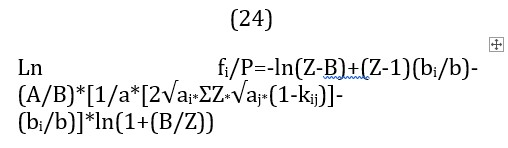PR state equation

The PR state equation was proposed by Peng and Robinson (1976) and is in fact a modified model of the Redlich-Kwong state equation to provide better vapor-liquid equilibrium calculations. The general form of the PR equation is defined based on the equation .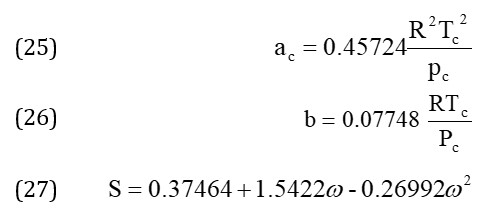Also, the compressibility coefficient is obtained by calculating the roots of the following equation.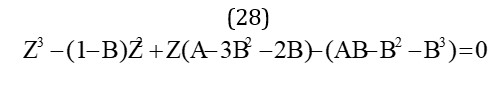The above equation has three roots, the least of which are related to the liquid phase and the most of which are related to the vapor phase, and the middle root has no special physical meaning. Now we can obtain fugacity with the following equation .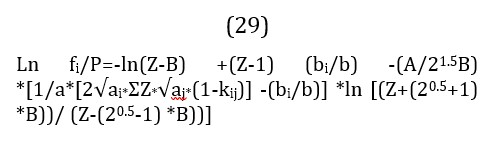Ln fi/P=-ln(Z-B) +(Z-1) (bi/b) -(A/21.5B) *[1/a*[2√ai*ƩZ*√aj*(1-kij)] -(bi/b)] *ln [(Z+(20.5+1) *B))/ (Z-(20.5-1) *B))]

PRSV state equation

The SRK and PR state equations are described in detail below. Regarding the PRSV state equation, the following explanations give a general reference to this state equation. The PRSV state equation, introduced in 1986, is a modification of the PR equation that increases the application of the original PR method to its non-ideal systems. The general form of the PRSV equation is defined based on the equation .

One of the modifications made by Stryjek and Vera to the PR equation is the use of an extended form α.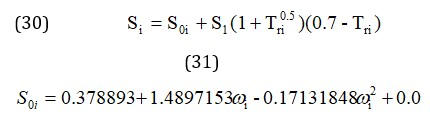The characteristic parameter and of the net component is the eccentricity coefficient of component i.

PT mode equation

The general form of the PT equation is defined based on the equation [59-61].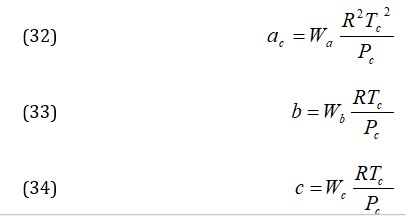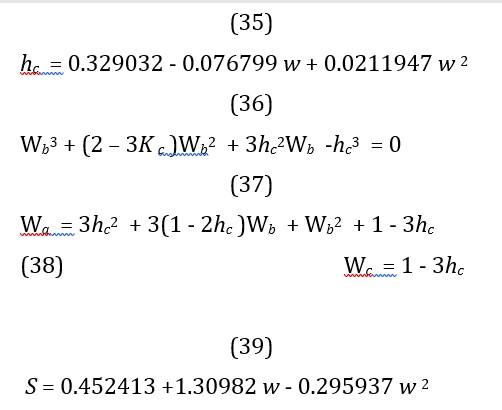S =0.452413+1.30982w-0.295937w2

Also, the compressibility coefficient is obtained by calculating the roots of the following equation.

The above equation has three roots, the least of which are related to the liquid phase and the most of which are related to the vapor phase, and the middle root has no special physical meaning .

Conclusion

For decades, gas hydrates have been a major problem in the oil and gas industry, and much attention has been paid to ways to prevent them from forming. For this reason, the prediction of hydrate formation conditions using thermodynamic models is well advanced and has good accuracy. However, since the use and applications of hydrate are less considered, less attention has been paid to production conditions and the factors affecting it, and only in the last two decades, studies on the kinetics of hydrate formation have been conducted. For the first time, Beniakas and Bisnovi (2020) presented a quasi-experimental model consistent with experimental results after studying the parameters affecting the kinetics of methane hydrate formation. Englerus et al. (2019) then investigated the kinetics of methane hydrate formation and proposed a new kinetic model considering gas fugacity and equilibrium fugacity as the driving force of hydrate formation. Lequam and Roof (2020) also used a kinetic model for the nucleation and growth process by providing a mechanism for the

formation of hydrates based on chemical reactions. In addition to research on the formation and decomposition of gas hydrates, the study of the effect of various additives on the formation of hydrates has also been of great interest to researchers. The synthesis of gaseous hydrate consists of two stages:

The first stage is nucleation inside the pores, the stage in which the molecules that make up the gaseous hydrate reach the pores where they become stable.

The second stage is the growth stage. After the first stage, nucleation, the hydrate crystals grow larger over time.

###### ##### References
 B. Raei, A. Bozorgian, Journal of Chemistry Letters, 2020, 1, 143-148. [crossref], [Google Scholar], [Publisher]
 S. Karami, M. Javan Nikkhah, KB. otouhifar, V. Rahjoo, A. Ahmadpour, Iranian Journal of Plant Protection Science, 2020, 51, 129-146. [crossref], [Google Scholar], [Publisher]
 M. Bagheri Sadr, A. Bozorgian, Journal of Chemical Reviews, 2021, 3, 66-82. [crossref], [Google Scholar], [Publisher]
 A. Haghighi Asl, A. Ahmadpour, N. Fallah, Journal of Modeling in Engineering, 2018, 16, 295-307. [crossref], [Google Scholar], [Publisher]
 A. Bozorgian, Journal of Chemical Reviews, 2021, 3, 50-65. [crossref], [Google Scholar], [Publisher]
 N. Kayedi, A. Samimi, M. Asgari Bajgirani, A. Bozorgian, South African Journal of Chemical Engineering, 2021, 35, 153-158. [crossref], [Google Scholar], [Publisher]
 S.V. Mousavi, A. Bozorgian, N. Mokhtari, M.A. Gabris, H.R. Nodeh, Microchemical Journal, 2019, 145, 914-920. [crossref], [Google Scholar], [Publisher]
 A. Bozorgian, Advanced Journal of Chemistry, Section B: Natural Products and Medical Chemistry, 2021, 3, 54-61. [crossref], [Google Scholar], [Publisher]
 A. Haghighi Asl, A. Ahmadpour, N. Fallah, Applied Chemistry, 2017, 12, 253-286. [crossref], [Google Scholar], [Publisher]
 A. Bozorgian, A. Samimi, International Journal of New Chemistry, 2021, 8, 41-58. [crossref], [Google Scholar], [Publisher]
 M. Bagheri sadr, A. Bozorgian, International Journal of Advanced Studies in Humanities and Social Science, 2020, 9, 252-261. [crossref], [Google Scholar], [Publisher]
 A. Bozorgian, Journal of Engineering in Industrial Research, 2020, 1, 1-19.  [crossref], [Google Scholar], [Publisher]
 A. Bozorgian, Progress in Chemical and Biochemical Research, 2020, 3, 169-179. [crossref], [Google Scholar], [Publisher]
 A. Surendar, A. Bozorgian, A. Maseleno, L.K. Ilyashenko, M. Najafi, Inorganic Chemistry Communications, 2018, 96, 206-210. [crossref], [Google Scholar], [Publisher]
 A. Bozorgian, Advanced Journal of Chemistry, Section B, 2020, 2, 91-101. [crossref], [Google Scholar], [Publisher]
 A. Bozorgian, Advanced Journal of Science and Engineering, 2020, 1, 34-39. [crossref], [Google Scholar], [Publisher]
 J. Mashhadizadeh, A. Bozorgian, A. Azimi, Eurasian Chemical Communication, 2020, 2, 536-547. [crossref], [Google Scholar], [Publisher]
 A. Bozorgian, International Journal of Advanced Studies in Humanities and Social Science, 2020, 9, 205-218. [crossref], [Google Scholar], [Publisher]
 M.E. Bidhendi, Z. Asadi, A. Bozorgian, A. Shahhoseini, M.A. Gabris, Environmental Progress & Sustainable Energy., 2020, 39, 13306. [crossref], [Google Scholar], [Publisher]
 A. Bozorgian, International Journal of Advanced Studies in Humanities and Social Science, 2020, 9, 241-251. [crossref], [Google Scholar], [Publisher]
 A. Bozorgian, International Journal of Advanced Studies in Humanities and Social Science, 2020, 9, 229-240. [crossref], [Google Scholar], [Publisher]
 A. Bozorgian, Chemical Review and Letters, 2020, 3, 94-97. [crossref], [Google Scholar], [Publisher]
 A. Bozorgian, Chemical Review and Letters, 2020, 3, 79-85. [crossref], [Google Scholar], [Publisher]
 A. Samimi, K. Kavosi, S. Zarinabadi, A. Bozorgian, Progress in Chemical and Biochemical Research, 2020, 3, 7-19. [crossref], [Google Scholar], [Publisher]
 A. Bozorgian, S. Zarinabadi, A. Samimi, Chemical Methodologies, 2020, 4, 477-493. [crossref], [Google Scholar], [Publisher]
. A. Bozorgian, Z.A. Aboosadi, A. Mohammadi, B. Honarvar, A. Azimi, Prog. Chem. and Biochem. Rese., 2020, 3, 31-38. [crossref], [Google Scholar], [Publisher]
 A. Bozorgian, Journal of Engineering in Industrial Research, 2021, 2, 90-94. [crossref], [Google Scholar], [Publisher]
 A. Bozorgian, Progress in Chemical and Biochemical Research, 2021, 4, 207-219. [crossref], [Google Scholar], [Publisher]
 A. Bozorgian, Z.A. Aboosadi, A. Mohammadi, B. Honarvar, A. Azimi, Journal of Chemical and Petroleum Engineering, 2020, 54, 73-81. [crossref], [Google Scholar], [Publisher]
 A. Pourabadeh, B. Nasrollahzadeh, R. Razavi, A. Bozorgian, M. Najafi, Journal of Structural Chemistry, 2018, 59, 1484-1491. [crossref], [Google Scholar], [Publisher]
 A. Bozorgian, Z. Arab Aboosadi, A. Mohammadi, B. Honarvar, A. Azimi, Eurasian Chemical Communications, 2020, 2, 420-426. [crossref], [Google Scholar], [Publisher]
 S.E. Mousavi, A. Bozorgian, International Journal of New Chemistry, 2020, 7, 195-219. [crossref], [Google Scholar], [Publisher]
 A. Bozorgian, S. Zarinabadi, A. Samimi, Journal of Chemical Reviews, 2020, 2, 122-129. [crossref], [Google Scholar], [Publisher]
 A. Samimi, S. Zarinabadi, A. Bozorgian, A. Amosoltani, Progress in Chemical and Biochemical Research, 2020, 3, 46-54. [crossref], [Google Scholar], [Publisher]
 B. Raei, A. Bozorgian, Journal of Chemistry Letters, 2021, 1, 143-148. [crossref], [Google Scholar], [Publisher]
 F. Zare Kazemabadi, A. Heydarinasab, A. Akbarzadeh, M. Ardjmand, Artificial cells, nanomedicine, and biotechnology, 2019, 47, 3222-3230. [crossref], [Google Scholar], [Publisher]
 F. Zare Kazemabadi, A. Heydarinasab, A. Akbarzadehkhiyavi, M. Ardjmand, Chemical Methodologies, 2021, 5, 135-152. [crossref], [Google Scholar], [Publisher]
 S. M. S. Mirnezami, F. Zare Kazemabadi, A. Heydarinasab, Progress in Chemical and Biochemical Research, 2021, 4, 191-206. [crossref], [Google Scholar], [Publisher]